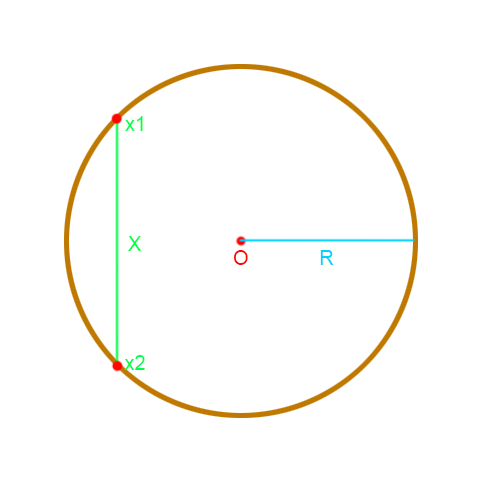# Getting two points' positions on a circleThe known factors are O (center of the circle), R (radius), and X’s position(and the distance between X and O naturally).

What I need to get here are the intersecting points of the straight line which always is perfectly vertical or horizontal. The circle is not a collider but just visual representation to show. Another fact in this is that X is always inside the range(within the radius) of the circle which means both x1 and x2 will always be at the radius distance from O.

Time to time I get really bummed out when trying to utilize known mathematical equations with coding and this is no different.

Simply trigonometric :

``````Vector2 x1 =  new Vector2 ((X - O), Mathf.Sqrt( Mathf.Pow(R,2) + Mathf.Pow((X - O) ,2)))

Vector2 x2 =  new Vector2 ((X - O), -Mathf.Sqrt( Mathf.Pow(R,2) + Mathf.Pow((X - O) ,2)))
``````

If x1 and x2 is at horizontal layout, reverse X and Y coordinate in “new Vector2”.

By courtesy of ‘Hellium’ at StackOverflow I was able to solve this quite easily. I also thank @x4637x for solving this as well.

If I understand your problem correctly,

X is a “random” point inside your
circle whose position is known : X =
(X.x, X.y).

Supposing you want to know X1 and X2
on the circle so that (X1X2) is a
vertical line. You know the absicca of
those points which is X.x.

You surely know that the coordinates
of a point on a circle can be defined
as : A = (O.x + r * cos θ, O.y + r *
sin θ) where :

O is the origin of the circle r is the

X1 = (O.x + r * cos θ1, O.y + r * sin
θ1) = (X.x, O.y + r * sin θ1) which
means

``````X.x = O.x + r * cos θ1 <=> cos θ1 = (X.x - O.x) / r <=> θ1 = arcos( (X.x - O.x) / r ) Once you know θ1, computing sin θ1 and X1.y is a piece
``````

of cake.

Computing X2 is very easy, you just
have to invert the ordinate.

If (X1X2) is an horizontal line, you
know the ordinates of X1 and X2 :

X1 = (O.x + r * cos θ1, O.y + r * sin
θ1) = (O.x + r * cos θ1, X.y)

``````X.y = O.y + r * sin θ1 <=> sin θ1 = (X.y - O.y) / r <=> θ1 = arcsin( (X.y - O.y) / r ) Here, to get X2 you
``````

have to invert the absicca.

His understanding of the question was on point and therefore I was able to do :

``````public IEnumerator FindRoute()
{
Vector3 O = attachedTarget.transform.parent.position;
Vector3 X = attachedTarget.transform.position;

float thetaOne;

{
thetaOne = Mathf.Asin((X.y - O.y) / maxRange);

pointA = new Vector3(O.x + (maxRange + 1) * Mathf.Cos(thetaOne), X.y, 0);
pointB = new Vector3(O.x + -((maxRange + 1) * Mathf.Cos(thetaOne)), X.y, 0);
yield return null;
}